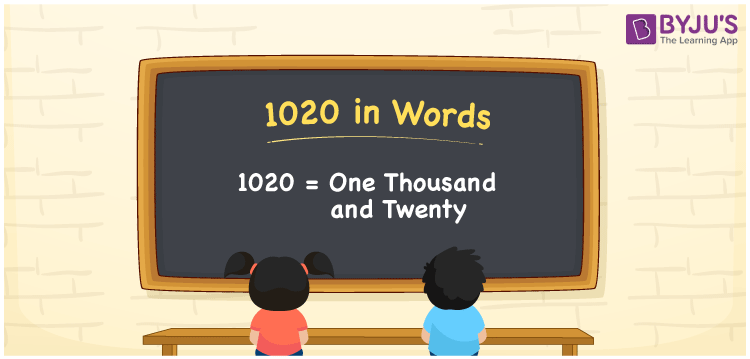# 1020 in Words

We can write 1020 in words as One Thousand and Twenty. Suppose you bought new specs that cost Rs. 1020, then you could say that “ I bought new specs for One Thousand and Twenty rupees”. In this article, you will learn how to convert the number 1020 into words in an exciting way. The number 1020 is used in expressions that relate to money, days, distance, length, weight and so on.

 1020 in words One Thousand and Twenty One Thousand and Twenty in Numbers 1020

## 1020 in English Words

We generally write numbers in words using the English alphabet. Thus, we can read 1020 in English as “One Thousand and Twenty”.## How to Write 1020 in Words?

In this section, you will learn how to write the number 1020 in words. The given number 1020 has four digits, so we should make a place value chart that represents the place value for all these four digits. The order of place values of digits in a number is in accordance with the Indian numbering system, such as:

• Ones
• Tens
• Hundreds
• Thousands
• Ten-thousands
• Hundred-thousands or Lakhs

This can be done as follows.

 Thousands Hundreds Tens Ones 1 0 2 0

Thus, we can write the expanded form as:

1 × Thousand + 0 × Hundred + 2 × Ten + 0 × One

= 1 × 1000 + 0 × 100 + 2 × 10 + 0 × 1

= 1020

= One thousand five hundred

1020 is a natural number that precedes 1021 and succeeds 1019.

1020 in words – One Thousand and Twenty

Is 1020 an odd number? – No

Is 1020 an even number? – Yes

Is 1020 a perfect square number? – No

Is 1020 a perfect cube number? – No

Is 1020 a prime number? – No

Is 1020 a composite number? – Yes

## Frequently Asked Questions on 1020 in Words

Q1

### How do you write 1020 in English?

We can write 1020 in English words as “One Thousand and Twenty”.
Q2

### How do you write 1020 in words for a cheque?

For a cheque, we generally write 1020 in words as One Thousand and Twenty rupees only.
Q3

### What number is 1020 in words?

The number 1020 is One Thousand and Twenty in words.